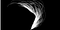One Of The hardest Math Problems That A Fifth Grader Can UnderstandThe image shows the paths of the first 10,000 numbers of the Collatz Conjecture sequence

The Collatz Conjecture, also known as 3n + 1 conjecture, Ulam Conjecture, Kakutani’s problem, Thwaites conjecture, Hasse’s algorithm or the Syracuse problem , is a opinion or conclusion formed on the basis of incomplete information in mathematics that no matter what value of a positive integer that we will call ‘n’, the sequence will always reach 1. The Collatz Conjecture is named after Lothar Collatz.

The sequence is defined as follows: start with any positive integer n. Then each term is obtained from the previous term as follows: if the previous term is even, the next term is one half the previous term. If the previous term is odd, the next term is 3 times the previous term plus 1. -Wikipedia

Mathematicians have tried over 2⁶⁰ integers which is almost 10²⁰ integer values, and all of them eventually reach the value 1. The algorithm is below.

`Collatz Conjecture Rules:  1. Start with any positive integer value 'n'  2. If the value is an even number then the next term is halved (n/2)  3. If the value is an odd number then the next term is that number times 3 plus 1 (3n+1)  4. Repeat this operation for the next term, and use that term as the new input(new positive integer value)`

# Collatz Conjecture Example

Start with n=6, the sequence will be 6, 3, 10, 5, 16, 8, 4, 2, 1.
To show this sequence n=6 and 6 is an even number so the next number will be 6/2 = 3. Now 3 is our new number and it is an odd number so the next number will be 3*(3) + 1 = 10. The number 10 is a even number so the next number in the sequence will be 10/2 = 5. The number 5 is an odd number so the next number will be 3*(5) + 1 = 16. The number 16 is an even number so the next number will be 16/2 = 8. The number 8 is an even number so the next number in the sequence will be 8/2 = 4. The number 4 is also even so the next number in the sequence will be 4/2 = 2. The number 2 is an even number so the next number will be 2/2 = 1.

Of course once we reach one we will start seeing a repeating value because 1 is an odd number the next number in the sequence will be 3(1) + 1 = 4, and we already see what sequence that produces. You can check out the video below and create a program using the Collatz Conjecture function to try it out yourself !

A copy of the C-Program from the video above can be found on my GitHub. If you can prove or disprove the Collatz Conjecture algorithm will always reach the value 1 given any positive integer, be sure to let me know in this article. To this day it has not been proven that this algorithm will always reach a value of 1 given any positive integer value.

Thanks for reading this article I hope its helpful to you all ! Keep up the learning, and if you would like more computer science, data structures, discrete mathematics and algorithm analysis videos please visit and subscribe to my YouTube channels (randerson112358 & compsci112358 )

Written by